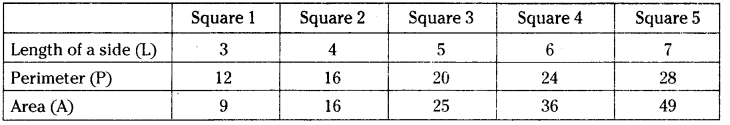# AP Board 7th Class Maths Solutions Chapter 6 Ratio – Applications Ex 3

AP State Syllabus AP Board 7th Class Maths Solutions Chapter 6 Ratio – Applications Ex 3 Textbook Questions and Answers.

## AP State Syllabus 7th Class Maths Solutions 6th Lesson Ratio – Applications Exercise 3Question 1.
A length of a bacteria enlarged 50,000 times attains a length of 5 cm. What is the actual length of the bacteria? If the length is enlarged 20,000 times only, what would be its enlarged length?
Solution:
After enlarging 50,000 times the length is 5 cm.
Without enlarging (1 – times) the length Is x cm say
∴ 50,000 : 5 :: 1 : x
By rule of properties
50,000 × x = 5 × 1
x = $$\frac{5}{50,000}=\frac{1}{10,000}$$ = 0.0001 cm

Question 2.
Observe the following tables and fmd if x is directly proportional.
Solution:
(i)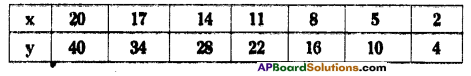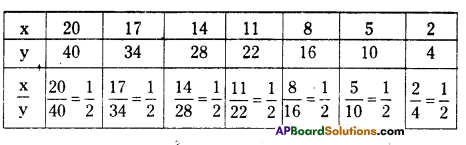In all the cases $$\frac { x }{ y }$$ is constant.
∴ x ∝ y (x is directly proportional toy)

(ii)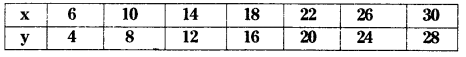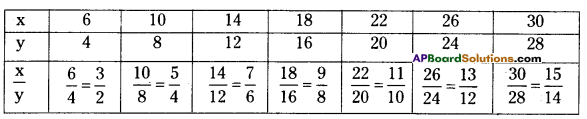Here the change/increase is not uniform.
(i.e.,) $$\frac { x }{ y }$$ is not same in all cases.
∴ x is not directly proportional to y.

(iii)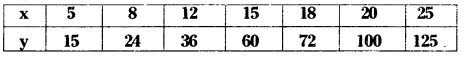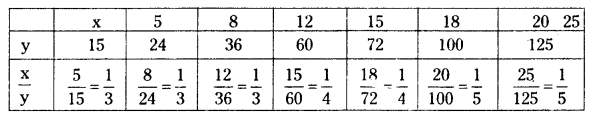Here the change/increase is not uniform.
(i.e.,) $$\frac { x }{ y }$$ is not same in all cases.
∴ x is not directly proportional to y.Question 3.
Sushma has a road map with a scale of 1 cm representing 18 km. She drives on a road for 72 km. What would be her distance covered in the map?
Solution:
Scale of the map is 1 cm = 18 km
Distance covered = 72 km
Let it be xcm on the map
then 1 : 18 :: x : 72
By rule of proportion
Product of extremes Product oÍ means
18x = 72 × 1
x = $$\frac{72}{18}$$ = 4cmQuestion 4.
On a Grid paper, draw five squares of different sizes. Write the following information in a tabular form.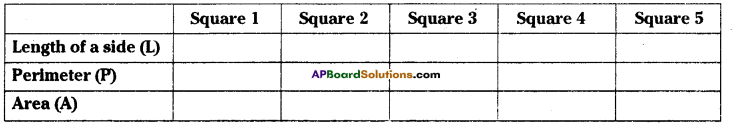Find whether the length of a side is in direct proportion to:
(i) the perimeter of the square.
(ii) the area of the square.
Solution: